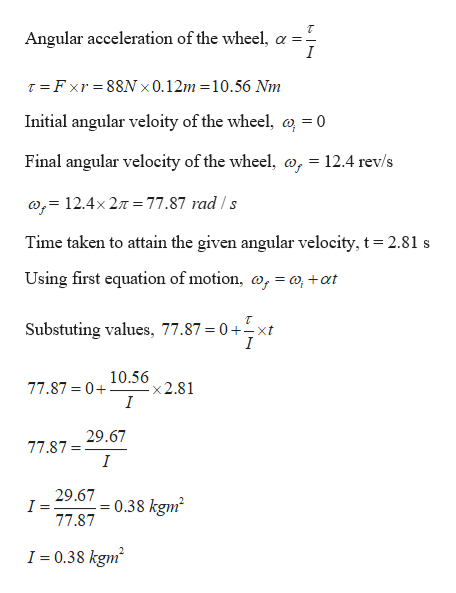# A wheel rotates without friction about a stationary horizontal axis at the center of the wheel. A constant tangential force equal to 88.0 N is applied to the rim of the wheel. The wheel has radius 0.120 m. Starting from rest, the wheel has an angular speed of 12.4 rev/s after 2.81 s.What is the moment of inertia of the wheel?

Question
1 views

A wheel rotates without friction about a stationary horizontal axis at the center of the wheel. A constant tangential force equal to 88.0 N is applied to the rim of the wheel. The wheel has radius 0.120 m. Starting from rest, the wheel has an angular speed of 12.4 rev/s after 2.81 s.

What is the moment of inertia of the wheel?

check_circle

Step 1

Given:

Force = 88 N

Radius of the wheel, r = 0.12 m

Step 2

Calculating the moment of i...help_outlineImage TranscriptioncloseAngular acceleration of the wheel, a - I T Fxr 88N 0.12m 10.56 Nm Initial angular veloity of the wheel, a = 0 Final angular velocity of the wheel, o, = 12.4 rev/s ,= 12.4x 27T= 77.87 rad/s Time taken to attain the given angular velocity, t = 2.81 s Using first equation of motion, o, = o+at Substuting values, 77.87 = 0+xt I 77.87 0 10.56 x2.81 I 77.8729.67 I 29.67 0.38 kgm 77.87 I 0.38 kgm fullscreen

### Want to see the full answer?

See Solution

#### Want to see this answer and more?

Solutions are written by subject experts who are available 24/7. Questions are typically answered within 1 hour.*

See Solution
*Response times may vary by subject and question.
Tagged in

### Rotational Mechanics